LC METER

Inductance  and Capacitance meter
using
"Frequency Shifted Oscillator"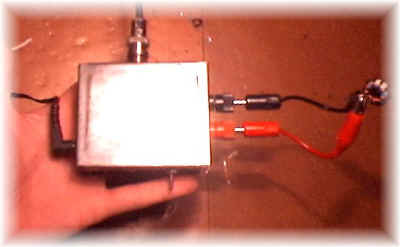The most mysterious components for radio-amateur (I suppose) are inductors;
infact you can measure all other (resistor capacitor) with a simple multimeter but not an inductor;
So you must:

1) pay a lot of money for a professional LC meter;
2) use other indirect, uncomfortable way;
3) go fishing HI.

I've tried  3) and 2), then I've  decided to home-made one.

I've built various L(C) meter; the following is one of best system but some more calculations are needed (who is the only disadvantage, so I've written a Visual Basic software to simplify operation).
It measure both inductance and capacitance (1-NanoHenry - 100 milliHenry for inductors and  1pF - 500nF for capacitor) and accuracy (who depends by calibration's component) is exellent,
but don't forget you're using a free oscillator, so frequency drift and inaccuracy at low value (1-10 nH, 1-10 pF) are to be expected;
With a lot of care in calibration, you can have exellent results.

Here follows a simple test where I compare LCMeter with my commercial Capacitance meter:

 Marked value Commercial C-meter LC Meter 10 pF 9,8 9,6 15 pF 14,9 14,6 100 Pf 99 99,6 2,2 nF 2,22 2,22 10 nF 9,96 10,11 100 nF 101 100,3

Regardig inductor, I can not make the same test because I have not a commercial L-Meter or well know inductor, but the same results are to be exected.

When I made the LC meter the first time, I've built it only as inductance meter; that was because I already have a commercial Capacitance meter and wanted avoid stray inductance-capactance due to switch, more cable etc, but there wasn't big differences so I've added back the C-meter.

The project is well know on the net because selled by AADE;
it works around a
"frequency shifted oscillator" who is well described in "RF Components and Circuit" by  RSGB;

Let's see schematic;
A LM311 oscillate at frequency F1 (determinated by L1 and C1, around 650 Khz);
There are 2 possible operating mode:

1) CX mode, where the unknow component is placed in parallel to L1 and C1 (and allows measuring both Inductance and capacitance) and

2) LX mode, who allows measuring only inductance placed in series with L1;

CX MODE

Placing C2 in parallel with L1 and C1 cause the LM311 to oscillate  at a lower frequency, F2 (the total capacitance is now C1+C2);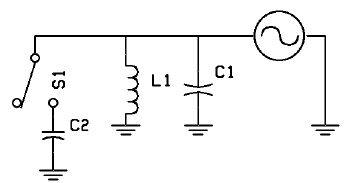If we know F1, F2, L1 e C1 we can calculate  C2 with a simple formula:
F1 ed F2 are well know  (we use a frequency-counter to measure it),  L1 and C1 are know only after calibrating the LC meter (wich is needed only once and is very easy to do).
We put a well know capacitor in parallel with C1 ;
frequency F1 will change in F2 (lower);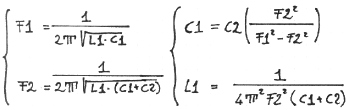F in Hertz   -  L in Henry  -   C in Farad
or
F in Khz   -  L in mH  -   C in mF
or
F in Mhz   -  L in uH  -   C in uF

solving the above equations we obtain C1 and L1 value, (stray inductance and capacitance are included);
now we know all we need, let's insert an unknow capacitor in parallel with L1,C1 ,measure the new frequency (F2) and calculate Cx :F in Hertz   -  L in Henry  -   C in Farad
or
F in Khz   -  L in mH  -   C in mF
or
F in Mhz   -  L in uH  -   C in uF

It's also possible measuring unknow inductance in CX mode; let's insert Lx in parallel to L1, C1.Calibration is the same as above (you don't need to re-make it), so just insert Lx, measure F2 and calculate Lx using the below formula: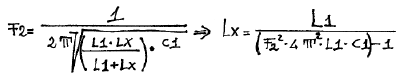F in Hertz   -  L in Henry  -   C in Farad
or
F in Khz   -  L in mH  -   C in mF
or
F in Mhz   -  L in uH  -   C in uF

LX MODE

Using an inductor (L2) in series with L1 cause the frequency to oscillate at F2 (lower than F1)  (when in series L total=L1+L2);Knowing  F1, F2, L1 e C1 it's possible calculate the unknow L2 with simple math formulas.

Infact F1 and F2 are well know, L1 and  C1 are know only after calibrating the LC Meter, calibration who  is very similar to CX mode but using different equations.
To calibrate the meter insert a well known inductor (L2) in series with L1;
F1 will change (down) to the new frequency F2;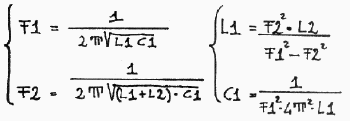F in Hertz   -  L in Henry  -   C in Farad
or
F in Khz   -  L in mH  -   C in mF
or
F in Mhz   -  L in uH  -   C in uF

Solving equations we obtain  C1 and L1 value, containing stray inductance and capacitance;

At this point we know all, just insert an unknow inductor (Lx), measure the new frequency (F2) and calculate Lx as below: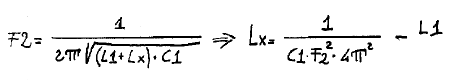F in Hertz   -  L in Henry  -   C in Farad
or
F in Khz   -  L in mH  -   C in mF
or
F in Mhz   -  L in uH  -   C in uF

CONSTRUCTION

I've used a small metallic box (8x6x3 cm), a bnc for connecting the frequency-counter, a power supply jack, a 2-way switch and test leads;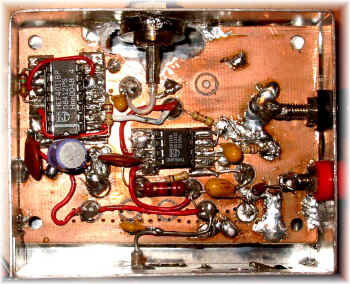A 1 Mhz frequency meter and calculator is needed ;
To avoid spending a lot of time in calculation I've written a Visual Basic software who  make all calculation pressing a key;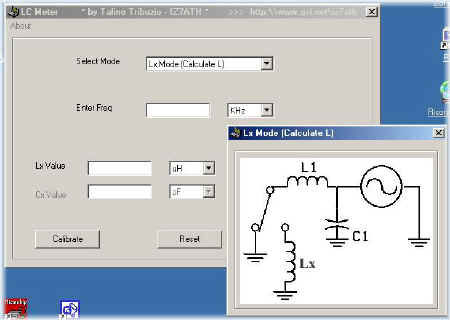USING LC METER

Switch-on LC Meter and wait few minutes;
Calibrate it  (CX mode calibration is better because you can easy-find  precise value capacitor, but you can calibrate it also in Lx mode using a well know inductor - anyway, however you calibrate it, results are ok for all mode) and read the new frequency (F2);
Insert F1, F2 C2 (or L2) in the software (or use the above formulas) to calculate  L1 and C1 (the tank circuit);
LC meter is now ready, just select the operating mode (LX o CX), insert unknow component and write F2 (the new frequency) in the software
: press F1 (or calculate button) and the calculated value will be shown on the screen.

If switching from Cx to Lx mode (in that case short-cut test leads) you read a different frequency (F1) don't worry, that's not a problem and is due to small stray inductance - capacitance; but that differences must be very small (i.e.: if i try to calculate Lx value short-cutting test leads with a very short wire, i obtain -4 / -11 nH);
If not so, re-wire  the circuit using as short as possible cable (expecially in the LC circuit).

Take wire as short as possible and calibrate LC meter more time during operations;
If accuracy measures are needed, don't use external test leads;
anyway using my software you can zeroing LC meter (only in LX mode, with the "Stray Inductance" textbox);
Just calibrate the meter (with no external test leads), switch to LX mode, insert and short-cut external test leads, calculate stray inductance and insert that value (in NanoHenry) in the "Stray Inductance" text box;
the software automatically will subtract that value in following measurements;

I've written some source code for a 16F84 PIC microcontroller to automatize all, but I've never finished it; I'll be happy to see that work finished.

P.S. in schematics I've added a LM7808 but you can use also 12V DC.